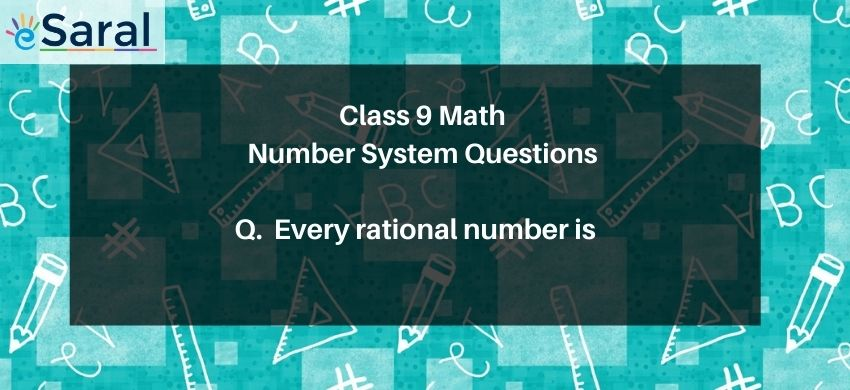Most Affordable JEE | NEET | 8,9,10 Preparation by Kota's Top IITian Doctor Faculties

# Every rational number is a real number# Question : Every rational number is

1. A natural number
2. An integer
3. A real number
4. A whole number
Solution :
The correct option is 3. A real number
Real number is a value that represents a quantity along the number line.
Real number includes all rational and irrational numbers.
Rational numbers are numbers that can be represented in the form $\frac{p}{q}$ where,
$\mathrm{q} \neq 0$ and $\mathrm{p}, \mathrm{q}$ are integers.
Therefore, a rational number is a subset of a real number.
We know that rational and irrational numbers taken together are known as real numbers. Therefore, every real number is either a rational number or an irrational number. Hence, every rational number is a real number. Therefore, (3) is the correct answer.